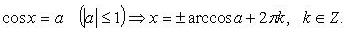# Answer to Question #5904 in Trigonometry for Shelby

Question #5904
cos(5theta - pie/6) = -square root 2/ 2
1
2012-02-14T11:47:18-0500
cos(5theta - pie/6) = -square root( 2)/ 2

we&#039;ll use the following formula for roots of such equation:so we obtain

5theta - pie/6=3*pie/4+2*pie*k or 5theta - pie/6=-3*pie/4+2*pie*k, where k is integer

Now we should find theta from obtained equations:

5theta =pie/6+3*pie/4+2*pie*k or 5theta =pie/6-3*pie/4+2*pie*k, where k is integer

5theta =11*pie/12+2*pie*k or 5theta =-7*pie/12+2*pie*k, where k is integer

theta =11*pie/60+(2/5)*pie*k or theta =-7*pie/60+(2/5)*pie*k, where k is integer - answer

Dear Shelby
For you and other our visitors we created this video. Please take a look!

Need a fast expert's response?

Submit order

and get a quick answer at the best price

for any assignment or question with DETAILED EXPLANATIONS!